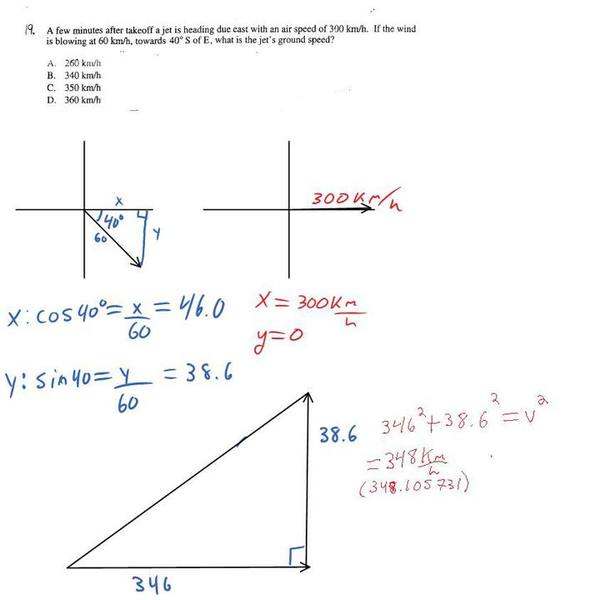Relative velocity

Last edited:

Homework Statement

http://i179.photobucket.com/albums/w282/jasonselinger/1234.jpg

The Attempt at a Solution

I got the answer 346, the real answer was 350. Did they just round up?

Thanks :)
$$|v| = \sqrt((300+60cos40)^2+(60 sin40)^2)$$